# Constituents Of The Atom

## Introduction

The atoms of all elements are made up of three particles called protons, neutrons and electrons. The protons and neutrons are at the centre of the atom. The centre of the atom is called a nucleus. The diameter of the nucleus is about 110000thof the diameter of the atom. In this article, these three elements and their properties are explained. The constituents of the atom are illustrated in Figure 1.Figure 1: Constituents of the Atom

A. Proton

A proton is a positively-charged particle found within the atomic nuclei. Protons are slightly smaller in mass than neutrons, with a relative mass of 0.9986 (as compared with the mass of the neutron being 1), or about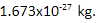The number of protons in an atom defines what element it is. For example, carbon atoms have six protons, hydrogen atoms have one, and oxygen atoms have eight.

The number of protons in an atom is referred to as the atomic number of that element. The number of protons in an atom also determines the chemical behaviour of the element. The Periodic Table of the Elements arranges elements in order of increasing atomic number.

B. Neutron

Neutrons are uncharged particles found within all atomic nuclei (except for hydrogen-1). A neutron’s mass is slightly larger than that of a proton. The neutron has a physical mass of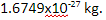C. Electron

Electrons are negatively charged tiny elements that orbit the nucleus of the atom. Electrons are 1,800 times smaller than either a proton or a neutron. Electrons have a relative mass of about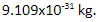D. Comparison of Charge and Mass

The following table summarises the charge and mass of all three elements of the atom.E. Atoms and Isotopes

The number of protons in the nucleus of an atom is called the proton number. It is also called an atomic number. A proton number is represented as Z. The number of protons together with neutrons in the nucleus is called the nucleon number. It is also called the mass number. Nucleon numbers are represented as A.

A particular atom, or a nuclide, of a chemical component Lithium with 3 protons and 4 neutrons is represented asIn general, a nuclide is represented as follows: The difference between nucleon number (A) and proton number (Z) of an atom gives the number of neutrons present in the nucleus.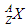Where, A – Nucleon number of an atom

Z – Proton number of an atom

X – Chemical symbol of an element.

Isotopes are different forms of the same element, which have the same number of protons but different numbers of neutrons in their nuclei. The following table shows the different isotopes of hydrogen.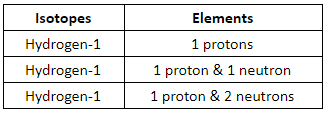F. Specific Charge

The specific charge of a particle or an atom is its charge to mass ratio. The unit of specific charge isWhere, Q – Charge of a particle or an atom (Coulomb)

m – Mass of a particle or an atom (kg).

Example Calculation: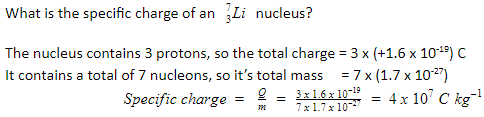G. Summary

• The atoms of all elements are made up of three particles called protons, neutrons and electrons.
• A proton is a positively-charged particle found within all atomic nuclei.
• Neutrons are uncharged particles found within all atomic nuclei.
• Electrons are negatively-charged tiny elements that orbit the nucleus of the atom.
• The number of protons in the nucleus of an atom is called the proton number or atomic number.
• The number of protons together with neutrons in the nucleus is called the nucleon number or mass number.
• The difference between a nuclear number and proton number of an atom gives the number of neutrons present in the nucleus.
• The specific charge of a particle or an atom is its charge to mass ratio. The unit of specific charge is